# Algebra - problems - page 61

1. PopulationWhat is the population of the city with 3% annual growth, if in 10 years the city will have 60,000 residents?
2. Geometric sequence 5About members of geometric sequence we know: ? ? Calculate a1 (first member) and q (common ratio or q-coefficient)
3. Family parcelsIn father will he divided the land so that the older son had three bigger part than younger son. Later elder son gave 2.5 ha field to younger and they had both the same. Determine the area of family parcel.
4. MO Z6-6-1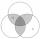Write integers greater than 1 to the blanks in the following figure, so that each darker box was product of the numbers in the neighboring lighter boxes. What number is in the middle box?
5. Pyramid Z8–I–6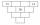Each brick of pyramid contains one number. Whenever possible, the number in each brick is lowest common multiple of two numbers of bricks lying directly above it. That number may be in the lowest brick? Determine all possibilities.
6. Diagonals of diamondFind the area and circumference of the diamond ABCD with 15m and 11m diagonals.
7. Cost reductionTwo MP3 players whose price was equal to originally have been discounted the first by 20%, the second by 35%. After the price reduction was the difference in their prices 750, - CZK. What was the original price of each of the two players?
8. How oldThe student who asked how many years he answered: "After 10 years I will be twice as old than as I was four years ago. How old is student?
9. Two numbersThe sum of the two numbers is 1. Find both numbers if you know that half of the first is equal to one seventh of the second number.
10. Cuboid edges in ratioCuboid edges lengths are in ratio 2:4:6. Calculate their lengths if you know that the cuboid volume is 24576 cm3.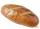5 of the same bread has the same weight as three bread and 4 kg of fruit. What weight has one bread?
12. Rabbits 3Viju has 40 chickens and rabbits. If in all there are 90 legs. How many rabbits are there with Viju?
13. Ruler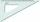How far from Peter stands 2m hight John? Petr is looking to John over ruler that keeps at arm's distant 60 cm from the eye and on the ruler John measured the height of 15 mm.
14. Two numbersWe have two numbers. Their sum is 140. One-fifth of the first number is equal to half the second number. Determine those unknown numbers.
15. Land - isosceles trapezoidCalculate the content and perimeter of the building plot in the form of an isosceles trapezoid with bases 120m, 95m and height 50m.
16. Unknown number 5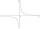Daniel think an integer. When he change this number at a ratio of 2:5 he got number 2.8. Determine what number think Daniel.
17. TreeBetween points A and B is 50m. From A we see a tree at an angle 18°. From point B we see the tree in three times bigger angle. How tall is a tree?
18. GlassTrader ordered from the manufacturer 200 cut glass. The manufacturer confirmed the order that the glass in boxes sent a kit containing either four or six glasses. Total sent 41 boxes. a) How many boxes will contain only 4 glasses? b) How many boxes will co
19. VCP equationSolve the following equation with variations, combinations and permutations: 4 V(2,x)-3 C(2,x+ 1) - x P(2) = 0
20. FactoryIn the factory workers work in three shifts. In the first inning operates half of all employees in the second inning and a third in the third inning 200 employees. How many employees work at the factory?

Do you have an interesting mathematical problem that you can't solve it? Enter it, and we can try to solve it.

To this e-mail address, we will reply solution; solved examples are also published here. Please enter e-mail correctly and check whether you don't have a full mailbox.